### Home > A2C > Chapter 4 > Lesson 4.2.4 > Problem4-122

4-122.
1. This is a Checkpoint for finding the slope of a line that passes through two points and for finding the distance between the points. Homework Help ✎Find the slope of a line that passes through each of the pairs of points given below and then find the distance between the two points.

1. (0, 0) and (4, 4)

2. (−2, 4) and (4, 7)

3. (12, 18) and (−16, −19)

4. (0, 0) and (25, 25)

5. Check your answers by referring to the Checkpoint 8 materials located at the back of your book.

If you needed help to find the slope or distance correctly, then you need more practice. Review the Checkpoint 8 materials and try the practice problems. Also, consider getting help outside of class time. From this point on, you will be expected to find slope and distance given two points easily and accurately.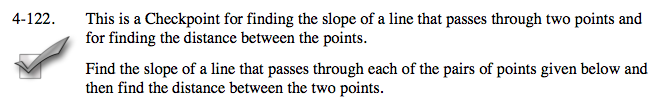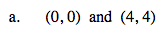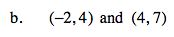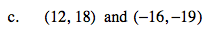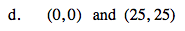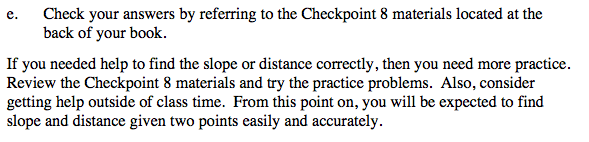Answers and extra practice are located in the back of your printed textbook or in the Reference Tab of your eBook.
If you have an eBook for A2C , login and then click the following link: Checkpoint 8 Answers and Practice